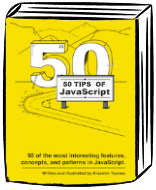My 5th book "50 Tips on JavaScript" is on sale. Go get it from https://50tips.dev.
Illustrated guide to 50 of the most interesting JavaScript topics.

# Meet sequence expression

You have no idea how fun is to transpiler JavaScript. I’m digging into that last few weeks and there is a step where I have to transform an AST into valid code. There is one tree node which I really like - sequence expression.

You are actually using sequence expression more often then you think. Here is an example:

``````(4, 8);
``````

The result of this line is `8`. We define different expressions which are evaluated from left to right. The result of the process is the result of the last one. In the example above that’s just the number `8`.

The sequence expression could be helpful in lots of cases and it is useful when the expressions are related to each other. Let’s check the following snippet:

``````var from = 0;
var to = 10;
var skipIf = function (value) {
return i === value ? (i = to, true) : false;
}
for(var i = from; skipIf(3), i < to; i++) {
console.log(i);
}
``````

The output is:

``````0
1
2
``````

There are two sequence expressions. The first one is in the condition bit of the `for` loop. The second one is part of the `return` statement in `skipIf` function. The idea of the code is to break the loop after the third iteration /For sure there are better ways to break a loop, but this example helps me illustrate the idea/

In `skipIf(3), i<to` we first run the function and then evaluate the condition. Both, the helper and the condition, are related to each other because the first one modifies a variable used in the second one. `skipIf` has two actions to perform if `i` matches the desire value. It should return `true` and it should stop the loop. `(i = to, true)` is doing exactly what we want. It changes `i` and returns the result of the second expression which is just `true`.

I started using sequence expression where I have to return a boolean based on some actions. For example:

``````function validate(item) {
if (42 === 42) {
item.status = 'valid';
}
return item;
}
var item = { status: null };
var isReady = (item = validate(item), item.status === 'valid');

if (isReady) { // true
// do something ...
}
``````

There is another type of expression which is widely used and it is close to sequence expression - logical expression. It also evaluates its parts from left to right but there are different rules applied.

``````var a = 'a';
var b = 'b';
(a && b); // returns b
(a || b); // returns a

var a = 0;
var b = 'b';
(a && b); // returns 0
(a || b); // returns b
``````

When we use `&&` the engine starts evaluating the expressions till it finds one that has falsy result. With `||` the engine continues till it finds truthy expression.

``````var a = 'a';
var b = 'b';
var c = 0;

(a && c && b); // returns 0
(c || a || b); // returns a
``````